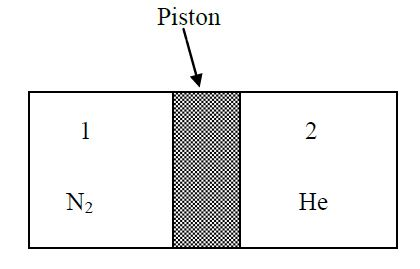# Gases in Equilibrium!

A thermally conducting piston can move freely in a thermally insulated cylindrical vessel, separating two compartments. Compartment $1$ contains $14mg$ of $N_2$ gas and compartment 2 contains $20mg$ of $He$ gas. When the piston attains its equilibrium position, the length of compartment $1$ becomes $L_1$ and that of compartment $2$ becomes $L_2$ (see figure). The molecular weight of nitrogen is $28$ and that of helium is $4$. Then the ratio is $\frac{L_1}{L_2}$×